Non-spherical particle shapes¶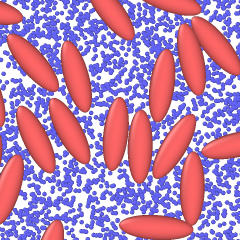Ellipsoidal particles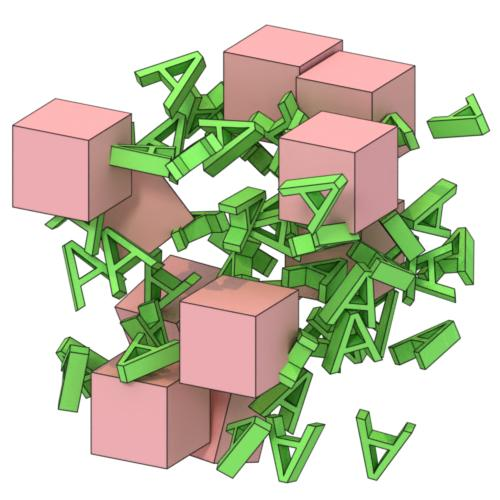User-defined particle shapes

OVITO has built-in support for a range of different particles shapes aside from the standard spherical shape. Furthermore, it supports user-defined particle shapes, which are specified in terms of polyhedral meshes:

• spheres

• ellipsoids

• cubes, boxes

• cylinders

• spherocylinders (capsules)

• circles (discs that follow the view direction)

• squares (billboards that follow the view direction)

• user-defined polygonal meshes

You can set the display shape of particles globally for the Particles visual element or on a per particle type basis in the Particle types panel.

Size and orientation of particles are controlled by properties¶

By assigning values to the following particle properties in OVITO, you can control the orientation and the dimensions or size of each particle individually:

• Aspherical Shape (X, Y, Z)

• Orientation (X, Y, Z, W)

The property assignment can happen directly during import of your data file into OVITO by mapping values from corresponding file columns to the right target properties in OVITO. Furthermore, you can subsequently assign values to these properties as needed by inserting the Compute property modifier into the a data pipeline.

The orientation of non-spherical particles is controlled by the Orientation particle property, which consists of four components $$\mathrm{q} = (x, y, z, w)$$ forming a quaternion. A quaternion is a mathematical representation of an arbitrary rotation in three-dimensional space, similar to (but more compact than) a rotation matrix.

Note that OVITO employs a notation for quaternions that follows the work of Ken Shoemake. This convention may slightly differ from other notations you find in the literature (e.g. on Wikipedia). The four quaternion components $$(x, y, z, w)$$ are sometimes interchanged and written as $$(q_r,q_i,q_j,q_k) = (w',x',y',z')$$ instead. Thus, during import of orientational data into OVITO, you may have to remap the four input components of the quaternions to the right target components.

The Orientation and Aspherical Shape particle properties are typically loaded from data files written by some simulation code. The LAMMPS simulation code, for example, allows you to output this per-particle information to a dump file using the following LAMMPS script commands:

compute orient all property/atom quati quatj quatk quatw
compute shape all property/atom shapex shapey shapez
dump 1 all custom 100 ellipsoid.dump id type x y z &
c_orient c_orient c_orient c_orient &
c_shape c_shape c_shape

You should map the quati, quatj, quatj, and quatw atom properties of LAMMPS to the Orientation.X, Orientation.Y, Orientation.Z, and Orientation.W properties of OVITO when importing the dump file.

Similarly, the shapex, shapey, and shapez columns need to be mapped to the properties Aspherical Shape.X, Aspherical Shape.Y, and Aspherical Shape.Z in OVITO if you are using one of the particle shapes described below where they play a role. Typically, you have to set up the mapping by hand in the Edit column mapping dialog, which is accessible from the file import panel after opening the dump or xyz file.

Spheres¶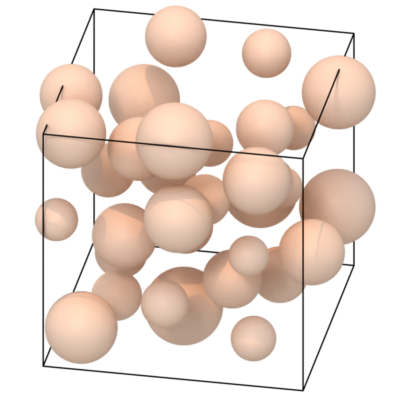The sphere particle shape is defined by the mathematical equation

$${\frac {x^2 + y^2 + z^2}{r^2}} = 1$$.

The sphere radius $$r$$ is controlled by the per-particle property Radius. If not present, the radius is determined by the particle type or, globally, by the Particles visual element.

The Position particle property specifies an additional translation of the spherical equation above away from coordinate system origin, of course.

Ellipsoids¶The ellipsoid particle shape is defined by the equation

$${\frac {x^2}{a^2}}+{\frac {y^2}{b^2}}+{\frac {z^2}{c^2}} = 1$$.

The length of the principal semi-axes $$a$$, $$b$$, $$c$$ of the ellipsoid are controlled by the per-particle property Aspherical Shape, which has three components X, Y, and Z (all positive). If all three components of the property are zero for a particle, OVITO falls back to $$a=b=c=r$$, with $$r$$ being the spherical radius of the particle as defined above.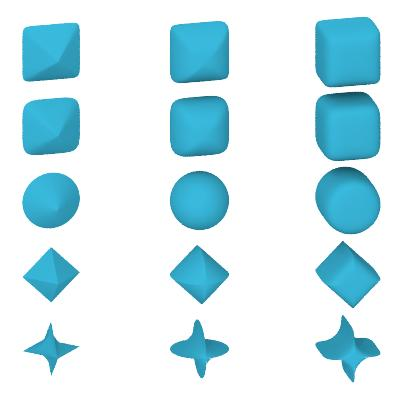The shape of superquadric particles is defined by the equation

$$\left( {\left| \frac{x}{a} \right| ^{(2/\phi)}} + {\left| \frac{y}{b} \right| ^{(2/\phi)}} \right) ^{(\phi/\theta)} + {\left| \frac{z}{c} \right| ^{(2/\theta)}} = 1$$.

Like ellipsoidal particles, the superquadric shape has three semi-axes $$a$$, $$b$$, $$c$$, which are specified by the Aspherical Shape particle property. The two parameters $$\phi$$ and $$\theta$$ are called east-west and north-south exponents and determine the blockiness/roundness of the superquadric ellipsoid. Both must be strictly positive. The normal sphere (or ellipsoid) is reproduced by setting $$\phi = \theta = 1$$. In OVITO, the values of $$\phi$$ and $$\theta$$ are specified by the Superquadric Roundness property, which is a vector particle property having two components.

Boxes¶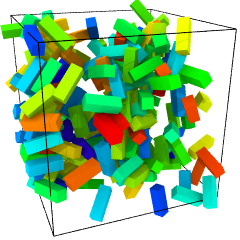The size of box-shaped particles is given by the semi-axes $$a$$, $$b$$, $$c$$, which are multiplied by a factor of two to yield the edge lengths of the box along the Cartesian coordinate axes. In OVITO the semi-axes are determined by the particle property Aspherical Shape, which has three components. If not present, or if the components of Aspherical Shape are zero for a particle, OVITO falls back to using the Radius particle property and renders a cube.

Cylinders¶

The cylindrical shape is given by the radius $$r$$ and the height $$h$$ (in the local coordinate system of the cylinder). The natural orientation of the cylinder is along the positive z-axis, with an optional rotation specified by the Orientation particle property. $$r$$ and $$h$$ are determined by the particle property components Aspherical Shape.X and Aspherical Shape.Z. The second vector component (Y) is ignored. If Aspherical Shape is not defined, OVITO will fall back to $$h = 2 r$$, with $$r$$ taken from the Radius property instead.

Spherocylinders (capsules)¶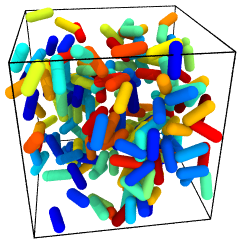The size of spherocylindrical particles is controlled in the same way as cylindrical particles. OVITO additionally render two hemispheres at each end of the cylinder, which extend the height of the cylinder.

Circles and squares¶

Circle and square are two-dimensional (i.e. flat) shapes, whose size is controlled by the Radius particle property. The orientation of each particle in three-dimensional space is determined automatically such that it exactly faces the viewer. Thus, their orientations are view-dependent and the Orientation particle property, if present, is ignored. In other words, you don’t have the possibility to control their orientations explicitly (use a mesh-based shape instead if you need control).

Note

Rendering of flat circles and squares is only possible with the OpenGL renderer of OVITO. The Tachyon and OSPRay rendering engines do not support this kind of particle shape.

User-defined shapes¶On the level of individual particle types you can assign custom particle shapes imported from external geometry files. OVITO supports loading general polyhedral meshes, which can serve as user-defined particle shapes, from various file input formats such as STL, OBJ, or VTK/VTP.

Some simulation formats such as HOOMD/GSD can embed information on particle shapes directly in the simulation output file, which will be picked up by OVITO automatically. In most cases, however, you’ll have to load the user-defined particle shape by hand for each particle type in the Particle types panel. Set the particle type’s Shape to Mesh/User-defined and import the shape geometry from a file that you have prepared outside of OVITO.

The vertex coordinates of the loaded polyhedral mesh get scaled by the value of the Radius property of each particle (if present) and rotated by the quaternion stored in the Orientation property (if present). Alternatively, you can set the Display radius parameter of the type to scale all particles of that type, or adjust the Radius scaling factor in the Particles visual element to scale all particles uniformly.